# Osculation

(diff) ← Older revision | Latest revision (diff) | Newer revision → (diff)

of a curvewith a curveat a given pointA geometrical concept, meaning thathas contact of maximal order withatin comparison with any curve in some given family of curves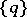including. The order of contact ofandis said to be equal toif the segment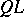is a variable of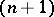-st order of smallness with respect to(see Fig., whereis perpendicular to the common tangent ofandat).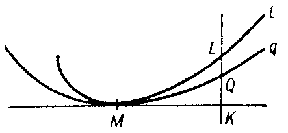Figure: o070590a

Thus, of all the curves in, the curve having osculation withis the one which is most closely adjacent to(that is, for whichhas maximal order of smallness). The curve inhaving osculation withat a given pointis called the osculating curve of the given family at this point. E.g., the osculating circle ofatis the circle having maximal order of contact withatin comparison with any other circle.

Similarly one can define the concept of osculation of a surfacein a given family of surfaceswith a curve(or with a surface) at some pointof it. Here the order of contact is defined similarly, except that one must examine the tangent plane ofatinstead of the tangent line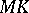in the figure.

How to Cite This Entry:
Osculation. Encyclopedia of Mathematics. URL: http://encyclopediaofmath.org/index.php?title=Osculation&oldid=15619
This article was adapted from an original article by BSE-3 (originator), which appeared in Encyclopedia of Mathematics - ISBN 1402006098. See original article